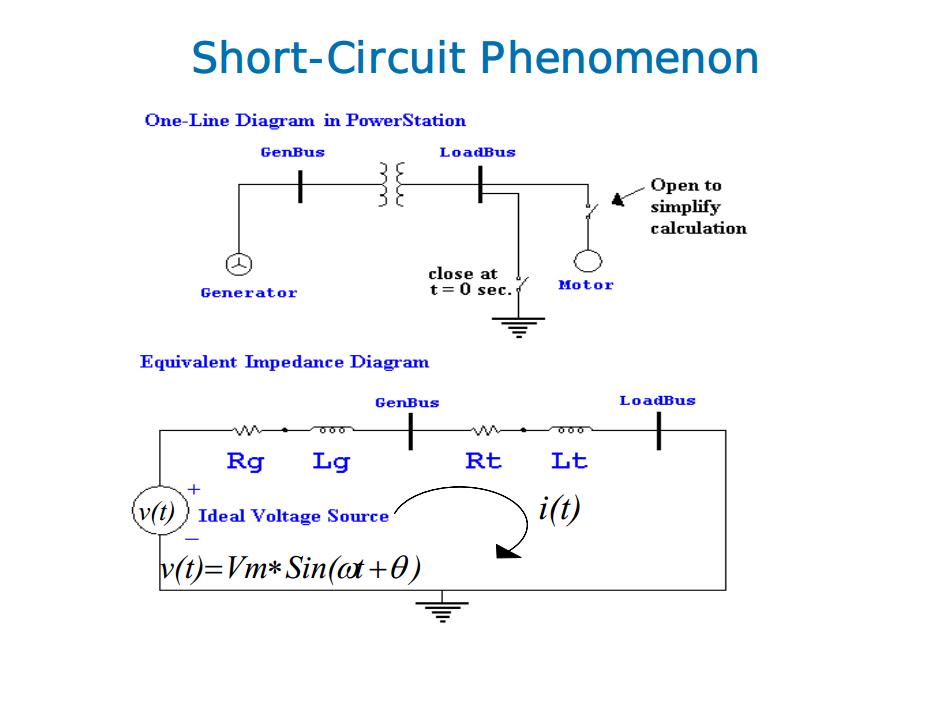# Advantages of Per Unit and Percent CalculationsPower System analysis software are very expensive beyond the reach of small and medium enterprises. The only option, when fault analysis is required, is to perform calculations manually using available resources such calculators or desktop computers. It maybe tedious but if it needs to be done, it has to be done.

Manual calculations can be MVA Method, Per unit and percent calculations. MVA Method of Calculation has been widely discussed in my previous articles. Per Unit and percent calculations simplify specification and computations particularly where different voltage levels and equipment sizes are involved.

Per unit and percent are similar. Percent is 100 times per unit. Both are used for convenience. It is important, however, to designate either percent (%) or per unit (pu). The per unit value of any quantity is the ratio of a quantity to its base value, the ratio expressed as a scalar decimal number. The actual quantities, such as voltage (V), current ( I ), power (P), reactive power (Q), volt-amperes (VA), resistance (R), reactance (X) and impedance (Z) can be expressed in per unit or percent as follows:where:
actual quantity = scalar or complex value of a quantity expressed in volts, amperes, ohms, watts, etc.
base value of quantity = an arbitrary or convenient reference of the same quantity chosen and designated as the base.

Thus per unit and percent are dimensionless ratios that may be either scalar or complex numbers.

Advantages of using per unit (or percent) are:

1. Results can be represented in magnitudes where all similar circuit quantities can be compared directly.
2. Equivalent impedance of any transformer is the same when referred to either the primary or the secondary side.
3. Impedance of a transformer in a three-phase system is the same regardless of the winding connections (wye-delta, delta-wye, wye-wye, or delta-delta)
4. Independent of voltage changes and phase shifts through transformers
5. Manufacturers specify the impedance of equipment in per unit or percent. The rated impedance can be used directly if the bases chosen are the same as the nameplate ratings
6. Per unit impedance of various ratings of equipment is only within a narrow range, while the actual ohmic values may vary widely. Where actual values are not known, a good approximate value can be used. Typical values for various types of equipment are available from many sources and reference books. Also, the correctness of a specified unit can be checked knowing the typical values.
7. Less chance of confusion between single-phase power and three-phase power, or between line-to-line voltage and line-to neutral voltage.
8. Very useful for simulating the steady-state and transient behavior of power systems on computers.
9. The drivingo r source voltage usually can be assumed to be 1.0 pu for fault and voltage calculations.
10. Product of two quantities expressed in per unit is expressed in per unit itself. However, the product of two quantities expressed in percent must be divided by 100 to obtain the result in percent. For this reason it is desirable to use per unit rather than percent in computations.

References:
Symmetrical Components fof Power Systems Engineering
J. Lewis Blackburn